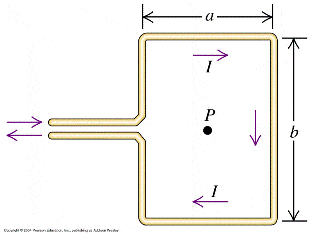Magnetic field from rectangular current loop

Another doozie for me. I have been trying this one for 3 days now. I will give my first born for help to this one :uhh:
---------------
Calculate the magnitude and direction of the magnetic field produced at point P in Fig. 28.52 by the current I in the rectangular loop. (Point P is at the center of the rectangle.) (Hint: The gap on the left-hand side where the wires enter and leave the rectangle is so small that this side of the rectangle can be taken to be a continuous wire with length b.)
Diagram-----------

I am using law of Biot and Savart(dB=(mu*I*dl x r)/(4pi*r^2))

I took the lines into four parts and started calculating what the magnetic field parts would be and then summ'ed them as by right hand rule the field will be into the page.

B1 is the left side, B2 is the top, B3 is the right and B4 is the bottom.

B3=B1=Mu*I*b/(Pi*a*sqrt(a^2+b^2))
B4=B2=Mu*I*a/(pi*b*sqrt(a^2+b^2))

Btotal=B4+B3+B2+B1=2Mu*I*b/(Pi*a*sqrt(a^2+b^2))+2Mu*I*a/(pi*b*sqrt(a^2+b^2))

Last edited by a moderator:

nrqed
Homework Helper
Gold Member
ovoleg said:
Another doozie for me. I have been trying this one for 3 days now. I will give my first born for help to this one :uhh:
---------------
Calculate the magnitude and direction of the magnetic field produced at point P in Fig. 28.52 by the current I in the rectangular loop. (Point P is at the center of the rectangle.) (Hint: The gap on the left-hand side where the wires enter and leave the rectangle is so small that this side of the rectangle can be taken to be a continuous wire with length b.)
Diagram-----------

I am using law of Biot and Savart(dB=(mu*I*dl x r)/(4pi*r^2))

I took the lines into four parts and started calculating what the magnetic field parts would be and then summ'ed them as by right hand rule the field will be into the page.

B1 is the left side, B2 is the top, B3 is the right and B4 is the bottom.

B3=B1=Mu*I*b/(Pi*a*sqrt(a^2+b^2))
B4=B2=Mu*I*a/(pi*b*sqrt(a^2+b^2))

Btotal=B4+B3+B2+B1=2Mu*I*b/(Pi*a*sqrt(a^2+b^2))+2Mu*I*a/(pi*b*sqrt(a^2+b^2))

You must *integrate* over the infinitesimal B fields produced by each little section of the wires. There is no wayto avoid using calculus here. Unfortunately I have to leave..hopefully someone will guide you through this

Last edited by a moderator:
I can only pray :(

I tried integrating along that wire.

Thanks anyone who can assist :)

Remember newborn might be ugly but he will work like a slave!

B1=mu*I*a/8*pi*Integral[ dy/((a/2)^2+y^2)^(3/2) ] from 0 to b

b1=b3=mu*I*b/(pi*a*sqrt(a^2+4b^2))

b2=b4 = mu*I*b/8pi*Integral[ dy/((a/2)^2+y^2)^(3/2) ] from 0 to a

b1+b2+b3+b4 = (2*mu_0*b)/(pi*a*sqrt(a^2+4b^2))+(2*mu_0*a)/(pi*b*sqrt(4a^2+b^2))

Anyone? I've looked through four physics books and I can't find a problem similar to this. The only thing I find is the one about the circle but the distance from point P is always the same(radius) so it doesn't help me one bit.

Extra love to anyone who can help :) must try to get done by tommorow afternoon

Last edited:
Anyone? :)

At this point I give up, anyone know an online resource I can find that will tell me the magnetic field generated by diffent types of loops?

I tried (2*mu_0*b)/(pi*a*sqrt(a^2+4b^2)) and (2*mu_0*a)/(pi*b*sqrt(4a^2+b^2)) seperately and they do not work

Anyone able to help out?

Alrighty I got it!!!!!!!!!!!!!!!!!!!! I am ummm, :zzz: :zzz: now but I got it done...

Okay for anyone that might have this come up again.

This is how you would do it.

1. First take each line as a segment and calculate the field from that line individually.

Using dB=mu*I*dl x r/(4pi*r^2) we can calculate this value

dB= mu*I*dl*sin(theta)/(4pi*r^2)

sin(theta) = x/(sqrt(x^2+y^2) (depending on the orientation then it obviously varies what you use for x, I just switches the axis around to make the problem simpler but you could definately have a defined axis and then proceed with the integration).

Since we can use the law of superposition in magnetism and magnetic fields, we calculate each on individually.

So evaluating the top and bottom bar with length a we will get

integral(dB)= mu*I*b/8pi * Integral( ((b/2)^2+y^2)^(3/2) from -a/2 to a/2)

evaluating the right hand integral we will get

B=mu*a/(b*pi*sqrt(a^2+b^2)) Remember b and I are constant in this integral so we take that outside of the integral.

B2=B4 = mu*a/(b*pi*sqrt(a^2+b^2))

Follow the same procedure for B1=B4 we will get

B= mu*b/(a*pi*sqrt(a^2+b^2))

and by right hand rule we will see that the field goes into the page from all of the lines so we would add up all the fields generated by each line segment(superposition) to find the magnetic field from the rectangular current loop.

The answer in all its glory : 2mu_0*I*b/(pi*a*sqrt(a^2+b^2)) + 2mu_0*I*a/(pi*b*sqrt(a^2+b^2))

http://www.webassign.net/www20/symImages/e/d/cf616f271f8a831a8ec00210690644.gif

Isn't it pretty?

Last edited by a moderator:
yes it is